## Sunday, June 29, 2008

### Sobel Edge Detection in Flash

The previous post Real Time Edge Detection in Flash shows Sobel edge detection and non-maxima suppression working on a live web cam feed. This post will quickly outline the steps required to build a Sobel edge detector in flash.
Sobel edge detection can be broken down into the following steps:
1. Greyscale the input image
2. Smooth the input image
3. Apply horizontal and vertical Sobel operators
4. Add the Sobel operators together to create an edge image.
An excellent example of this method can be found here. This implementation can be greatly increased in speed as will be shown.

### SetupFirst need to set up the bitmapData objects which will store the intermediate and final results, these will all be the size of the inputImage
``private var inputImage:BitmapData;private var greyScaleImage: BitmapData = new BitmapData(inputImage.width, inputImage.height);private var blurredImage: BitmapData = new BitmapData(inputImage.width, inputImage.height);private var horizontalEdge:BitmapData= new BitmapData(inputImage.width, inputImage.height, true, 0xff000000);private var verticalEdge:BitmapData= new BitmapData(inputImage.width, inputImage.height, true, 0xff000000);private var outputEdge:BitmapData= new BitmapData(inputImage.width, inputImage.height, true, 0xff000000);``

### Greyscale Input Image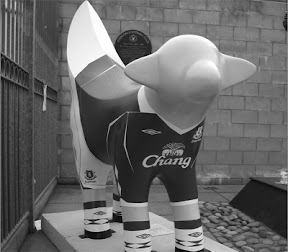There are a number of different ways to convert a colour image to greyscale, it really doesn't matter which one we choose too much. For this reason a simple greyscale will be used, which averages the three colour channels into one channel. The average of the red, green and blue colour channels will be put on the blue colour channel, this will lead to a 'bluescale' image, which will be used for speed. Flash uses the ColourMatrixFilter object to apply a colour transform (more information here).

``public const third:Number = 1 / 3;public const blueScaleArray:Array = [0, 0, 0, 0, 0,                                     0, 0, 0, 0, 0,                                     third, third, third, 0, 0,                                     0, 0, 0, 1, 0];public const blueScaleFilter:ColorMatrixFilter = new ColorMatrixFilter(blueScaleArray);greyScaleImage.applyFilter(inputImage, inputImage.rect, new Point(), blueScaleFilter);``

### Gaussian Blur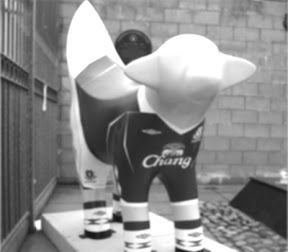A Gaussian is used to smooth the image to reduce noise, larger Gaussians smooth the image to a greater degree. Using the convolution filter a 3x3 or 5x5 Gaussian can be made. The notes from Adobe show that a 3x3 filter within certain limits will work significantly faster on most computers than other filters.
``private const GAUSSIAN_3BY3:ConvolutionFilter = new ConvolutionFilter(3,3,[ 1,2,1, 2,4,2, 1,2,1], 16);blurredImage.applyFilter(greyScaleImage, greyScaleImage.rect, new Point(), GAUSSIAN_3BY3);``

### Sobel Operators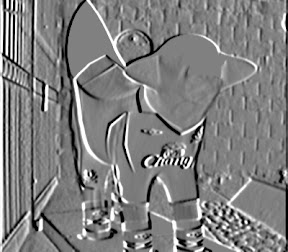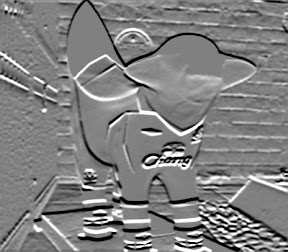After the image is smoothed horizontal and vertical Sobel operators are applied to the image to create two separate images (again using the convolution filter). The Sobel operators create two edge images, one showing the horizontal edge responses and the other the vertical. The convolution filter is offset by 127 so that both positive (white to black) and negative (black to white) edge responses can be stored in the image. Sobel is analogous to a first derivative function; it records where an abrupt change in grey-level occurs: an edge.
``private const VERTICAL_SOBEL:ConvolutionFilter = new ConvolutionFilter(3,3,  [ -1, 0, 1,   -2, 0, 2,   -1, 0, 1], 1, 127);      private const HORIZONTAL_SOBEL:ConvolutionFilter = new ConvolutionFilter(3,3,  [ -1, -2, -1,   0, 0, 0,   1, 2, 1], 1, 127);horizontalEdge.applyFilter(blurredImage, blurredImage.rect, new Point(), HORIZONTAL_SOBEL);verticalEdge.applyFilter(blurredImage, blurredImage.rect, new Point(), VERTICAL_SOBEL);``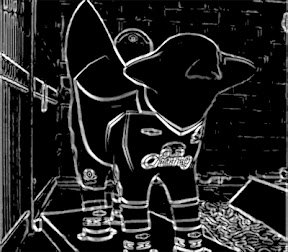The gradient magnitude image is the combination of both the Sobel edge images. Since there is an offset of 127 on the edge images and both negative and positive edge responses are recorded each pixel of the 2 edge images must be iterated over and combined correctly. There are two ways to achieve this (as shown in the Real Time Edge Detection in Flash demo). The first method takes advantage of Flash's built in bitmapData methods but is less accurate, the second is slower but more accurate. In Both methods a threshold can be set, between 0 and (0xff /2), to determine the minimum edge strength which appears in the output image.

#### Method 1

Both edge images are thresholded and added together, using the methods BitmapData.theshold and BitmapData.draw using Blendmode.ADD to add the two thresholded images together. There are a number of approximations made with this method: the negative edges are discarded and the positive edges are added, but the result is reasonable.
``outputEdge.threshold(horizontalEdge, horizontalEdge.rect, new Point(), ">", threshold,0,0xff,true);verticalEdge.threshold(verticalEdge, verticalEdge.rect, new Point(), ">", threshold, 0, 0xff, true);outputEdge.draw(verticalEdge, null, null, BlendMode.ADD);``

#### Method 2

In this method two thresholds are used: lower and upper, the upper threshold is simply (0xff - lowerThreshold). Each blue pixel of both edge images are read in (just the blue channel is used because red=green=blue in a greyscale image). The pixels are then tested to see if they exceed the lower or upper thresholds (positive and negative edge thresholds). The 127 offset is then removed from the pixel so that the edge responses are now positive and negative. The gradient magnitude image in theory is the route of the sum of the square of both the edge responses, this is very slow to compute, so a good approximation is to sum the absolute value of both numbers. The computed value is then converted back from just a blue pixel value to a grey value and set in the edge image.
``//--intermediate results storagevar currentHorInt:int;var currentVerInt:int;var currentHorUint:uint;var currentVerUint:uint;var value:uint;for ( var y:int = 0; y < outputEdge.height; y++){ for (var x:int = 0; x < outputEdge.width; x++) {       //---just use the blue channel---//  currentHorUint = horizontalEdge.getPixel(x,y) & 0xff ;   currentVerUint = verticalEdge.getPixel(x, y) & 0xff ;        //swap OR for AND for speed  if (!(currentHorUint > _lowerThreshold && currentHorUint < _upperThreshold && currentVerUint > _lowerThreshold && currentVerUint <_upperThreshold))  {         //use just blue channel and convert so that they can have positive or negative values by removing 127 offset   currentHorInt = currentHorUint - 127;   currentVerInt = currentVerUint - 127;   //write gradient magnitude minus one because otherwise the max value is 256      value = uint(Math.abs(currentHorInt) + Math.abs(currentVerInt)) - 1;         //-- greyscale instead of bluescale   value = (value << 16) + (value << 8) + value;   outputEdge.setPixel(x,y, value );        } }}``

## Optimising

There are a number of optimisations which can be made, I found the following increased execution time most: (testing on a 1.8 Ghz AMD with 1 Gb RAM with an input image 1024x1280 pixels)

1. using a 3x3 Gaussian over a 5x5 (saved half a second)

2. making function scoped copies of values before looping. This optimisation really surprised me, making local copies class scoped variables and statics before looping massively increased performance

3. Using a bluescale instead of greyscale

Further speed improvements can be made by not greyscaling or blurring the image, although this will technically give an inferior edge it is often hard to see the difference with the eye. The reason the image is inferior is that the edge is being created just from the blue channel of the input image, which isn't necessarily representative of the other 2 channels and there will be more noise in the image, this can be overcome to some degree by setting a lower threshold value. Below is a comparison of the 2 edge images: with blurring and greyscale (left) without (right).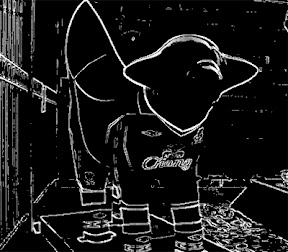Hope you enjoyed the post.

Labels: , ,Carl Looper said...

Another way of combining the H and V components is to use the BitmapData.paletteMap method to map values where you want them. And use the BitmapData.merge method to combine the results.

10:19 PMwal5hy said...

thanks Carl, I haven't used that method before will have to give it a try

11:06 PMAnonymous said...

i already try your method to do detection ,but the result is not good. could u giva me the value of low and high threshold for my reference thanks a lot

4:59 PMLawrie said...

Pretty cool. I saw this recently, which seems to be doing a very good job too - http://blog.inspirit.ru/?p=297

3:19 PMJoe Hocking said...

This looks great, thanks for explaining your methodology! Any chance you could post source for a working example? I tried to put your code into my project and it isn't working right, so I need to know what I did wrong.

6:07 PM